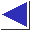# comp.lang.c FAQ list ·Question 13.19

Q: How can I return a sequence of random numbers which don't repeat at all?

A: What you're looking for is often called a ``random permutation'' or ``shuffle.'' One way is to initialize an array with the values to be shuffled, then randomly interchange each of the cells with another one later in the array:

```	int a, i, nvalues = 10;

for(i = 0; i < nvalues; i++)
a[i] = i + 1;

for(i = 0; i < nvalues-1; i++) {
int c = randrange(nvalues-i);
int t = a[i]; a[i] = a[i+c]; a[i+c] = t;	/* swap */
}
```
where randrange(N) is rand() / (RAND_MAX/(N) + 1) or one of the other expressions from question 13.16.

References: Knuth Sec. 3.4.2 pp. 137-8